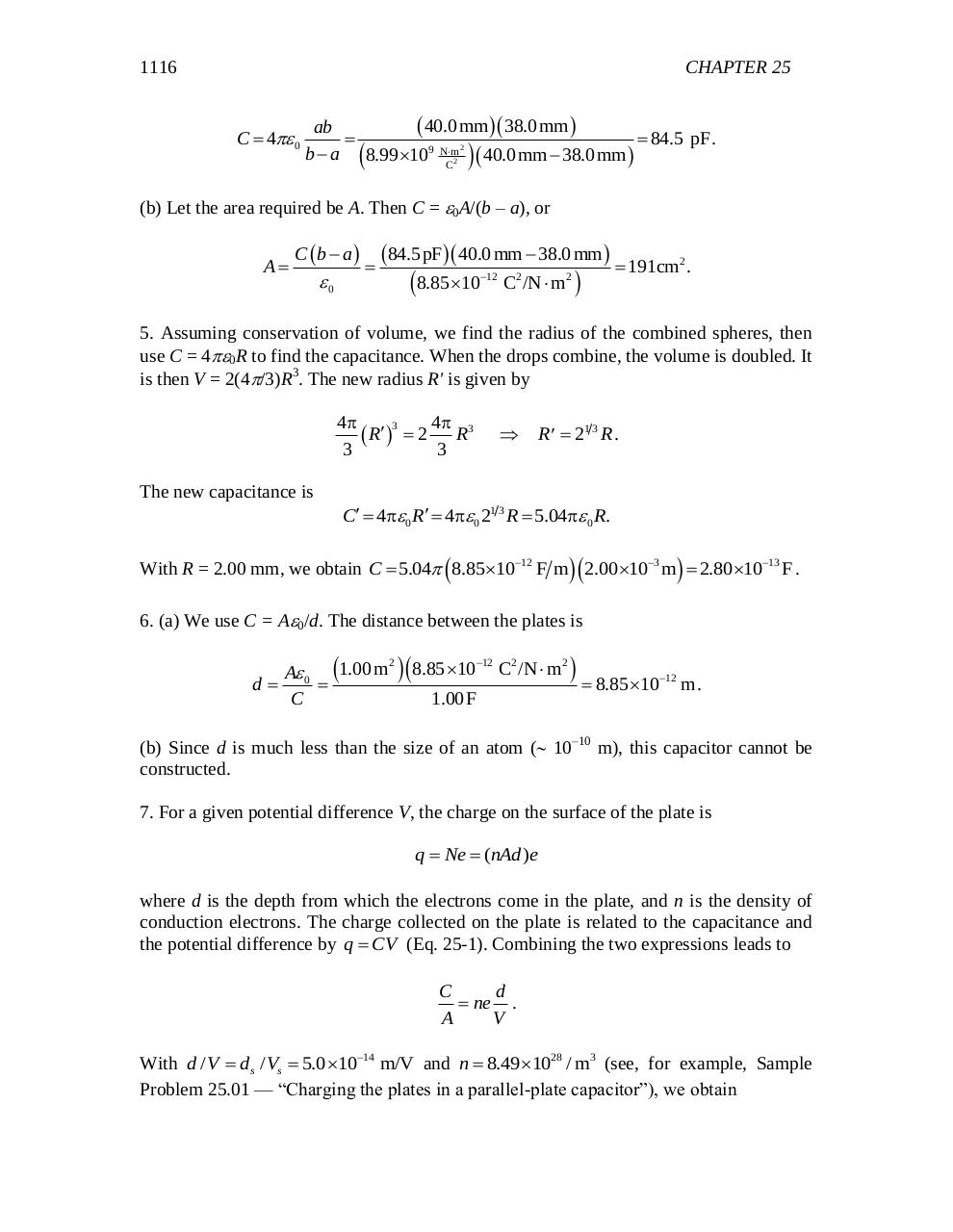# chapter 25.pdfPage 1 23432

#### Text preview

1116

CHAPTER 25

C  4 0

 40.0 mm  38.0 mm 
ab

 84.5 pF.
b  a 8.99 109 Nm2 2  40.0 mm  38.0 mm 
C

(b) Let the area required be A. Then C = 0A/(b – a), or

A

C b  a 

0

84.5pF 40.0 mm  38.0 mm   191cm2 .

8.85 10

12

C2 /N  m2 

5. Assuming conservation of volume, we find the radius of the combined spheres, then
use C = 40R to find the capacitance. When the drops combine, the volume is doubled. It
is then V = 2(4/3)R3. The new radius R' is given by
4p
4p
3
 R  2 R3
3
3

R  21 3 R .

The new capacitance is
C  4p 0 R  4p 0 21 3 R  5.04p 0 R.

With R = 2.00 mm, we obtain C  5.04 8.851012 F m  2.00 103 m   2.80 1013 F .
6. (a) We use C = A0/d. The distance between the plates is

d

A 0 1.00 m

C

2

8.85 10

12

C2 /N  m2 

1.00 F

 8.85 1012 m.

(b) Since d is much less than the size of an atom ( 10–10 m), this capacitor cannot be
constructed.
7. For a given potential difference V, the charge on the surface of the plate is
q  Ne  (nAd )e

where d is the depth from which the electrons come in the plate, and n is the density of
conduction electrons. The charge collected on the plate is related to the capacitance and
the potential difference by q  CV (Eq. 25-1). Combining the two expressions leads to
C
d
 ne .
A
V

With d / V  ds / Vs  5.0 1014 m/V and n  8.49 1028 / m3 (see, for example, Sample
Problem 25.01 — “Charging the plates in a parallel-plate capacitor”), we obtain